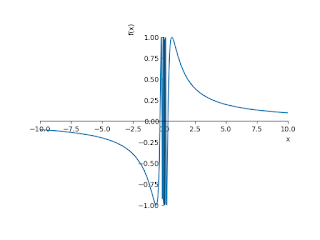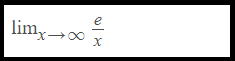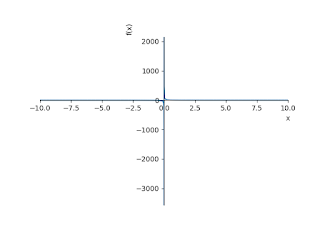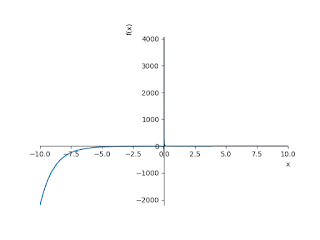## The limit of a function as x tends to infinity

In this unit, we explain what it means when the Limit of a function tends to infinity, at least infinity, or to a true bond, while x tends to infinity or infinity. We also explain what it means that a function tends to be real, while x tends to a certain real number.

In any case, we provide an example of a function that does not reach a limit. To master the techniques described here, it is important that you do many exercises to make it second nature.

In this example, we use Python programming to check the limit when x goes to infinity. The limit is a value that a function "approaches" because the input approaches some value. Limits are integral to calculus and are defined by using continuity, derivatives, and integrals.

In this article various python libraries used. Now Let's take a look at how to find the limit for the given function when x tends to infinity in Python code.

## Examples Limit x tends to infinity:1. Discuss the limit of the following function when x→∞
```import numpy as np
from sympy import *
x = symbols('x')
init_printing(use_unicode = True)
print(limit (sin(1/x),x,oo,'+'))
plot(sin(1/x))
Output: 0The limit of a function as x tends to infinity

```
2. Discuss the limit of the following function when x→∞```import numpy as np
from sympy import *
x = symbols('x')
init_printing(use_unicode = True)
print(limit (exp(1)/x,x,oo,'+'))
plot(exp(1)/x)
Output: 0
```The limit of a function as x tends to infinity
```
```
3. Discuss the limit of the following function when x→∞```import numpy as np
from sympy import *
x = symbols('x')
init_printing(use_unicode = True)
print(limit (exp(-1)/x,x,oo))
plot(exp(-1)/x)
Output: 0
```
```The limit of a function as x tends to infinity

```
```4. Discuss the limit of the following function when x→∞import numpy as np
from sympy import *
x = symbols('x')
init_printing(use_unicode = True)
print(limit (exp(x)/x,x,oo))
plot(exp(x)/x)
Output: ooThe limit of a function as x tends to infinity

5. Discuss the limit of the following function when x→∞import numpy as np
from sympy import *
x = symbols('x')
init_printing(use_unicode = True)
print(limit (exp(-x)/x,x,oo))
plot(exp(-x)/x))The limit of a function as x tends to infinity

6. Discuss the limit of the following function when x→∞import numpy as np
from sympy import *
x = symbols('x')
init_printing(use_unicode = True)
print(limit (x**2*sin(1/x),x,oo,'+'))
plot(x**2*sin(1/x))
Output: ooThe limit of a function as x tends to infinity

7. Discuss the limit of the following function when x→∞import numpy as np
from sympy import *
x = symbols('x')
init_printing(use_unicode = True)
print(limit (x/(1+x),x,oo,'+'))
plot(x/(1+x))
Output: 1The limit of a function as x tends to infinity

```